# 相量图和相量代数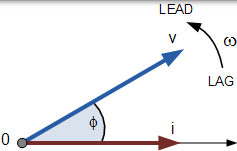### 正弦波形的相量图### 正弦波形的相位差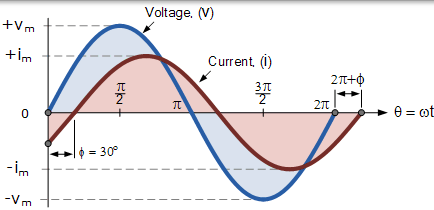$$\begin{array} { l } { \mathrm { V } _ { ( \mathrm { t } ) } = V _ { \mathrm { m } } \sin ( \omega \mathrm { t } ) } \\ { \mathrm { i } _ { ( \mathrm { t } ) } = \mathrm { I } _ { \mathrm { m } } \sin ( \omega \mathrm { t } - \phi ) } \end{array}$$

### 正弦波形的相量图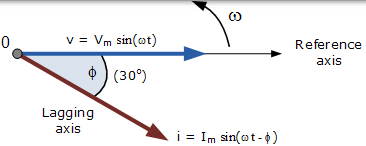## 相量相加

### 两个相量的相量加法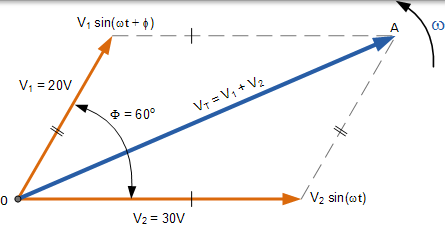### 复杂正弦波的定义

$$V _ { m } = \cos ( \phi ) + j V _ { m } ( \sin \phi )$$

$$\begin{array} { l l } { A = x + j y } & { B = w + j z } \\ { A + B = ( x + w ) + j ( y + z ) } \end{array}$$

## 使用矩形形式的相量加法

• 水平分量 = 30 cos 0o = 30 伏
• 垂直分量 = 30 sin 0o = 0 伏

• 水平分量 = 20 cos 60o = 20 x 0.5 = 10 伏
• 垂直分量 = 20 sin 60o = 20 x 0.866 = 17.32 伏

• 然后，这给出了电压 V1 的矩形表达式 ： 10 + j17.32

• VHorizontal = V1 和 V2 的实部之和 = 30 + 10 = 40 伏
• VVertical = V1 和 V2 的虚部之和 = 0 + 17.32 = 17.32 伏

$$\mathrm { V } _ { \mathrm { T } } = \sqrt { 实部 ^ { 2 } + 虚部 ^ { 2 } } \\ \mathrm { V } _ { \mathrm { T } } = \sqrt { 40 ^ { 2 } + 17.32 ^ { 2 } }$$

### VT 的 结果值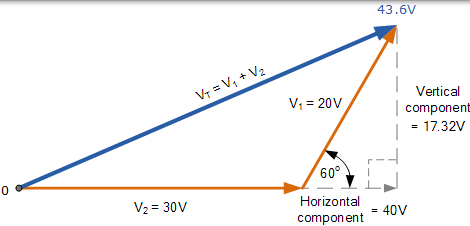## 相量减法

### 两个相量的矢量减法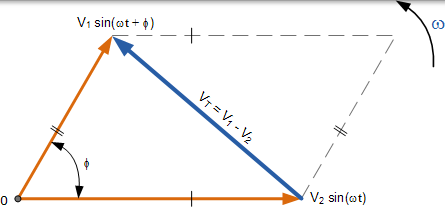$$\begin{array} { l l } { A = x + j y } & { B = w + j z } \\ { A - B = ( x - w ) + j ( y - z ) } \end{array}$$

## 三相相量图

### 三相相量图### 三相电压方程

\begin{aligned} \text { RedPhase: } & \mathrm { V } _ { \mathrm { RN } } = \mathrm { V } _ { \mathrm { m } } \sin \theta \\ \text { Yellow Phase: } & \mathrm { V } _ { \mathrm { YN } } = \mathrm { V } _ { \mathrm { m } } \sin \left( \theta - 120 ^ { \circ } \right) \\ \text { Blue Phase: } \mathrm { V } _ { \mathrm { BN } } & = \mathrm { V } _ { \mathrm { m } } \sin \left( \theta - 240 ^ { \circ } \right) \\ or, \mathrm { V } _ { \mathrm { BN } } & = \mathrm { V } _ { \mathrm { m } } \sin \left( \theta + 120 ^ { \circ } \right) \end{aligned}

## 相量图摘要

• 矢量，相量相量图仅适用于正弦交流交流量。
• 相量图可用于在任何时刻表示两个或更多个固定的正弦量。
• 通常，参考相量沿水平轴绘制，并且在该时刻绘制其他相量。所有相量均参考水平零轴绘制。
• 可以绘制相量图来表示两个以上的正弦曲线。它们可以是电压，电流或其他一些交变量，但它们的频率必须相同
• 所有相量都沿逆时针方向旋转。据说参考相量之前的所有相量都是超前，而参考相量后面的所有相量都被称为滞后
• 通常，相量的长度表示正弦量的 rms 值而不是其最大值。
• 由于矢量的速度不同，不同频率的正弦波不能在相同的相量图上表示。在任何时刻，它们之间的相位角将是不同的。
• 可以将两个或更多个向量一起添加或减去，并成为单个向量，称为结果向量
• 向量的水平边等于实数或 x 向量。向量的垂直边等于虚数或 y 向量。合成的直角三角形的斜边等同于合成向量。
• 在三相平衡系统中，每个相位移相 120°